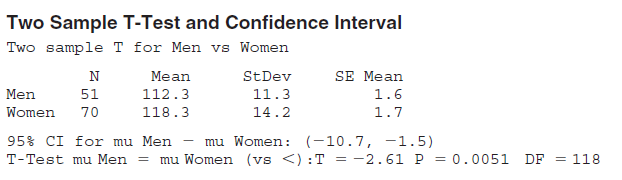×
Get Full Access to Statistics: Informed Decisions Using Data - 5 Edition - Chapter 11.3 - Problem 21
Get Full Access to Statistics: Informed Decisions Using Data - 5 Edition - Chapter 11.3 - Problem 21

×

# ?Comparing Step Pulses A physical therapist wanted to know whether the mean step pulse of men was less than the mean step pulse of women. She randomlyISBN: 9780134133539 240

## Solution for problem 21 Chapter 11.3

Statistics: Informed Decisions Using Data | 5th Edition

• Textbook Solutions
• 2901 Step-by-step solutions solved by professors and subject experts
• Get 24/7 help from StudySoup virtual teaching assistantsStatistics: Informed Decisions Using Data | 5th Edition

4 5 1 347 Reviews
29
3
Problem 21

Comparing Step Pulses A physical therapist wanted to know whether the mean step pulse of men was less than the mean step pulse of women. She randomly selected 51 men and 70 women to participate in the study. Each subject was required to step up and down onto a 6-inch platform for 3 minutes. The pulse of each subject (in beats per minute) was then recorded. After the data were entered into Minitab, the following results were obtained(a) State the null and alternative hypotheses.

(b) Identify the P-value and state the researcher’s conclusion if the level of significance was= 0.01.

(c) What is the 95% confidence interval for the mean difference in pulse rates of men versus women? Interpret this interval.

Step-by-Step Solution:

Step 1 of 5) Comparing Step Pulses A physical therapist wanted to know whether the mean step pulse of men was less than the mean step pulse of women. She randomly selected 51 men and 70 women to participate in the study. Each subject was required to step up and down onto a 6-inch platform for 3 minutes. The pulse of each subject (in beats per minute) was then recorded. After the data were entered into Minitab, the following results were obtained (a) State the null and alternative hypotheses. (b) Identify the P-value and state the researcher’s conclusion if the level of significance was = 0.01. (c) What is the 95% confidence interval for the mean difference in pulse rates of men versus women Interpret this interval. For example, we obtain a simple random sample of individuals and ask them to disclose their number of years of education.

Step 2 of 2

##### ISBN: 9780134133539

This full solution covers the following key subjects: . This expansive textbook survival guide covers 88 chapters, and 2422 solutions. Since the solution to 21 from 11.3 chapter was answered, more than 226 students have viewed the full step-by-step answer. Statistics: Informed Decisions Using Data was written by and is associated to the ISBN: 9780134133539. This textbook survival guide was created for the textbook: Statistics: Informed Decisions Using Data, edition: 5. The answer to “?Comparing Step Pulses A physical therapist wanted to know whether the mean step pulse of men was less than the mean step pulse of women. She randomly selected 51 men and 70 women to participate in the study. Each subject was required to step up and down onto a 6-inch platform for 3 minutes. The pulse of each subject (in beats per minute) was then recorded. After the data were entered into Minitab, the following results were obtained (a) State the null and alternative hypotheses.(b) Identify the P-value and state the researcher’s conclusion if the level of significance was = 0.01.(c) What is the 95% confidence interval for the mean difference in pulse rates of men versus women? Interpret this interval.” is broken down into a number of easy to follow steps, and 121 words. The full step-by-step solution to problem: 21 from chapter: 11.3 was answered by , our top Statistics solution expert on 01/15/18, 03:19PM.

## Discover and learn what students are asking

Unlock Textbook Solution